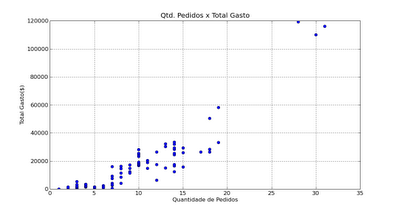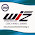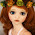## Sunday, August 23, 2009

Hello Folks!

In this article we will start a deep study about Algorithms used at Data Mining. I will explain how to use the classic classification algorithm (clustering) for data segmentation in accordance to categories called K-Means Clustering Algorithm. One simple version of the algorithm will be shown here implemented with Python, similar to the other articles posted here at this blog.

The Algorithm
The main idea from the K-Means algorithm is to provide the classification of a lot of information based on its own data. This classification, that it will be shown next, is based on analysis and comparison between numerical values from the data. Thus, the algorithm automatically will provide a autonomous classification without human supervision, that is, with no existing classification. Because of this characteristic, the K-Means is considered as an unsupervised data mining algorithm.
To understand how the algorithm works, let's imagine that we have a table distributed with lines and columns that contains a lot of samples to be classified. In this table, each column is called of dimension and each line contains information for each dimension, which can be also called of ocurrences or dots. Generally, this algorithm works with continously samples, but it can also treat discrete data, provided that they must be mapped to corresponding numerical values.
As i said earlier, the algorithm will analyse all the samples of this table and generate clusters (classifications). So, the algorithm will classify the data into one cluster and indicate which lines (patterns) belong to this cluster (class). The user or the developer must provide to the algorithm the number of clusters (k) that the data must be partitioned. This number of clusters (K) remembers the first letter of the algorithm: K-means.

To generate the clusters and classify the samples, the algorithm makes a comparison between each value of the line based on a distance measure. Generally, it's used the euclidian distance to calculate how "far" the attribute of the pattern is from each other. How to evaluate this distance depends on how many attributes exist from the provided table. After the calculation of the distances, the algorithm computes the centroid for each one of the clusters. While the algorithm goes through each step, the value of each centroid is recomputed based on the mean of the values of each attribute of each pattern that belongs to this centroid. Thus, the algorithm results with k centroids and put the paterrns of the table in accordance to its distance of centroids.
To simplify all the explanation of how the algorithm works, i will present the K-means process at the following steps:
Step 01: Begin with a decision on the value of k = number of clusters.
In this step, the k centroids must be initiated. You may assign the training samples randomly, or systematically as the following:
1. Take the first k training samples of the table as single-element clusters
2. Assign each of the remaining (N-k) training samples to the cluster with the nearest centroid. After each assignment, recomputed the centroid of the gaining cluster.
Step 02: Create a distance matrix between each pattern and the centroids.
In this step, for each sample in sequence compute its distance from the centroid of each of the clusters. The drawback of this step is the heavy calculation, since we have N samples and k centroids, the algorithm will have to evaluate NxK distances.
Step 03: Put each sample in the cluster with the closest centroid (minimal distance).
Here, the samples are classified based on its distance from the centroid of each of the clusters. If a sample is not currently in the cluster with the closest centroid, switch this sample to that cluster. Notice that the algorithm will end when no data is moving to another cluster anymore.
Step 04: Update the new centroids for each cluster.
At this moment, the centroid location is updated. For each centroid of the cluster that gained or lost a sample, its location is updated through calculating the mean of each attribute of all samples that belong to the respective cluster.
Step 05: Repeat until the convergence condition satisfied.
The algorithm comes back to the Step 02 , repeating the adjustment process of the location of each centroid until convergence is achieved, that is until a pass through the training sample causes no new assignments.
The fluxogram of all steps described above can be ilustrated at the Figure 01 below.
Figure 01. K-means Algorithm Process
One can note that we will have a classification that puts each sample at only one cluster. Thus, we can conclude that this algorithm generates a "hard clustering," once each sample can only be classified at only one class. Other algorithms work with the "soft" classification concept, where there is one metric that measures the degree of the sample belong to each class.
If you want to read more about the algorithm K-Means, you can look further at the link below and even download other implementations of the algorithm:
Now that we introduced the algorithm, let's see a practical example using the K-means technique.
Practical Example of the use of K-means
In this example, let's suppose a company that sells products to clients by orders made up of a list of itens (products). To make easy the comprehension of the problem and the data scenary, we will consider objects ( X clients) and each object have two attributes or features as shown in Figure o2 below.Figure 02. Data Set samples
Based on this model, the marketing department desires to segment the clients to offer exclusive discounts and other benefits. Our goal is to group these clients of the marketing data set into three categories: Gold , Silver and Bronze Clients. The client classification criteria must consider only the two attributes: the total of orders of each client and the total cost of the client in his all orders without discounts. Obviously that the clients that have more orders and with higher total costs will be classified as Golden Clients.
With the all objects shown at the table at the Figure 2, each client will be represented as one point with two attributes (X,Y) where X = Number of Orders and Y = Total Cost. The Figure 02 shows the chart based on those attributes mapped into coordinates.
We will use the algorithm K-means to classify the data set in accordance to the marketing department wants. As it was only specified two attributes (Total Cost and number of orders) , those will be used to classify the clients. In real problems the algorithm K-Means could also work with any number of attributes to classify the objects.
Analyzing the data of the Figure 03, we can predict that the three clients will be classified as Golden Clients, therefore it's easy to see the distance between these clients and the others. However it's not so easy the classification of the rest of the clients into Silver and Bronze Clients categories.Figure 03. Scatter Plot (Total of Orders x Total Cost)

To help the classification of these clients, we will use a implementation of the K-means algorithm that will work with only two attributes. This implementation in Python named k_means.py and can be seen here.
To execute the algorithm we the module at the console with the following parameters:
%% python k_means.py <"dataSet pathFile">
EX: %%python k_means.py "C:/dataSet.txt" 3
Running the algorithm with the data set we presented earlier, the results were very satisfactory. In our example, the K-means classified the data into three classes: class 1, 2 and 3. Based on the definition of the client type, we associate the class 1 to Bronze Client, the class 2 to Silver Client and class 3 to the Golden Client. Putting these samples at scatter plot, we can visualize clearly the classification of the clients. This chart is shown at the Figure 04.
Interpreting the chart presented at the Figure 04, the clients represented by the color green are the Golden Clients, the clients at color red are the Silver Clients and the clients at blue color are the Bronze Clients. The three triangles in yellow point are the centroids calculated by the algorithm.Figure 04. Clustered Data after running the K-means algorithm.
With the use of the K-means algorithm, it's possible to classify the current clients in accordance to their number of orders and the total cost in all orders, as the company marketing department desired. To classify a new client, just execute again the implementation and verify which is its classification. Thus, all clients will be again analyzed and classified.
One future feature could also be implemented is compare the attributes of a new client to the centroids ones before including it at the data set. This comparison is done by calculating the distance between the values of the new client and values of all centroids provided by the algorithm. Thus, the new client is said belong to the cluster that has minimum distance from this data.
PS: To run completely showing the charts presented above, you must have MatplotLib and Python installed at your PC. It's necessary for plotting the charts.
I expect that you enjoyed learning about some algorithms used at the Data Mining process. Wait for more tutorials soon!
See you next time,
Marcel

1.the Requested file is deleted... :S where can i get it????

thanks

2.Solved the problem , sorry for the delay.

3.Embedded system training: Wiztech Automation Provides Excellent training in embedded system training in Chennai - IEEE Projects - Mechanical projects in Chennai Wiztech provide 100% practical training, Individual focus, Free Accommodation, Placement for top companies. The study also includes standard microcontrollers such as Intel 8051, PIC, AVR, ARM, ARMCotex, Arduino etc.

Embedded system training in chennai
Embedded Course training in chennai
Matlab training in chennai
Android training in chennai
LabVIEW training in chennai
Arduino training in chennai
Robotics training in chennai
Oracle training in chennai
Final year projects in chennai
Mechanical projects in chennai
ece projects in chennai

4.WIZTECH Automation, Anna Nagar, Chennai, has earned reputation offering the best automation training in Chennai in the field of industrial automation. Flexible timings, hands-on-experience, 100% practical. The candidates are given enhanced job oriented practical training in all major brands of PLCs (AB, Keyence, ABB, GE-FANUC, OMRON, DELTA, SIEMENS, MITSUBISHI, SCHNEIDER, and MESSUNG)

PLC training in chennai
Automation training in chennai
Best plc training in chennai
PLC SCADA training in chennai
Process automation training in chennai
Final year eee projects in chennai
VLSI training in chennai

5.6.Well done! It is so well written and interactive. Keep writing such brilliant piece of work. Glad i came across this post. Last night even i saw similar wonderful R Programming tutorial on youtube so you can check that too for more detailed knowledge on R Programming.https://www.youtube.com/watch?v=gXb9ZKwx29U

7.I think things like this are really interesting. I absolutely love to find unique places like this. It really looks super creepy though!!
Best Machine Learning institute in Chennai | Best Machine learning training | best machine learning training certification